Dr. J's Maths.com
Where the techniques of Maths
are explained in simple terms.

Trigonometry - auxilliary angles.
Test Yourself 1 - solutions.

 1.2.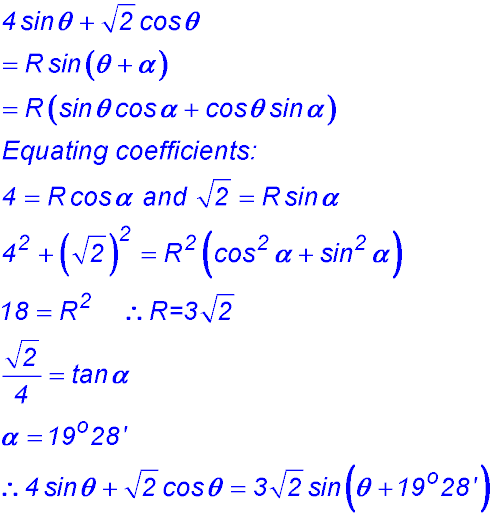. 3. (i)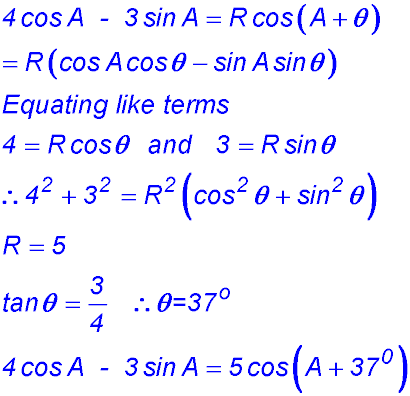(ii)4.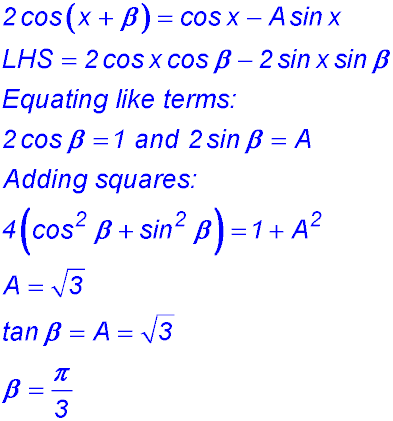5.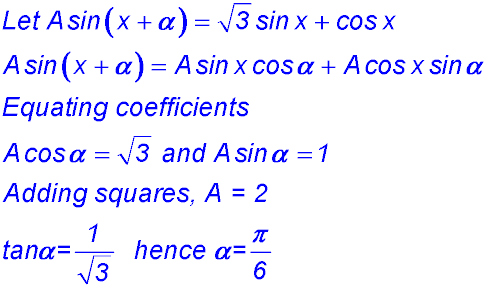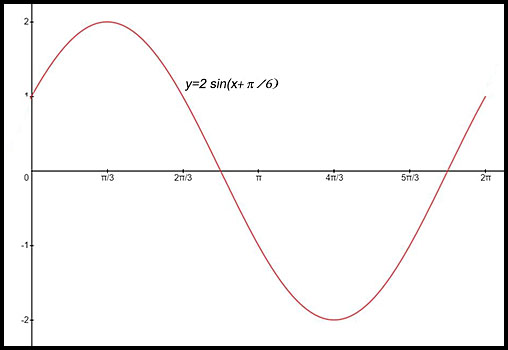6. (i)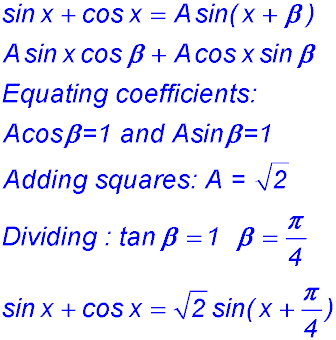(ii) Hence sketch y = sin x + cos x (for -π ≤ x ≤ 2π)?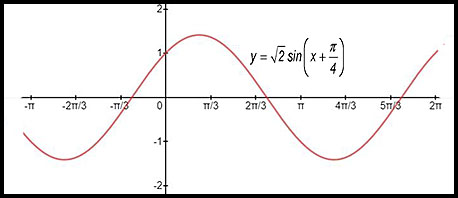(iii) How many solutions are there for sin x + cos x = 1 within that domain? As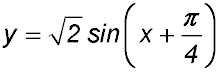= 1 at x = 2π (due to the horizontal shift), there are three solutions to the equation. 7.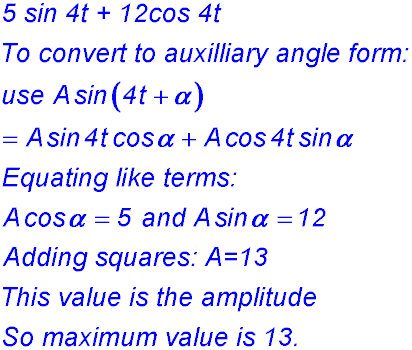8. (i)(ii) Sketch the curve for 0° ≤ x ≤ 360° .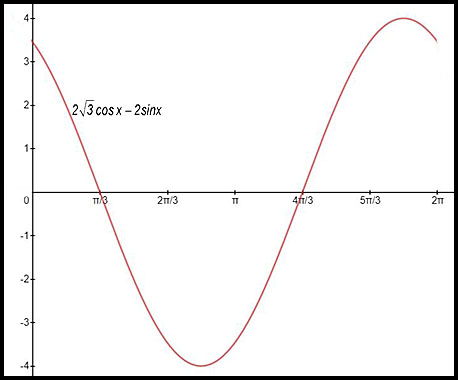9. (i)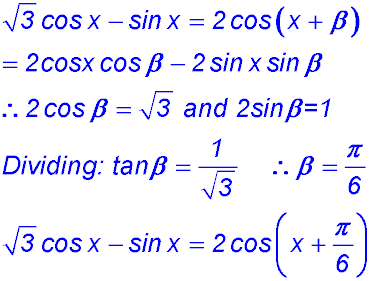(ii) Hence or otherwise solve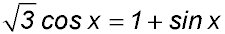for 0 ≤ β ≤ 2π.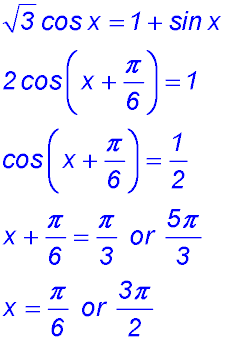10. (i)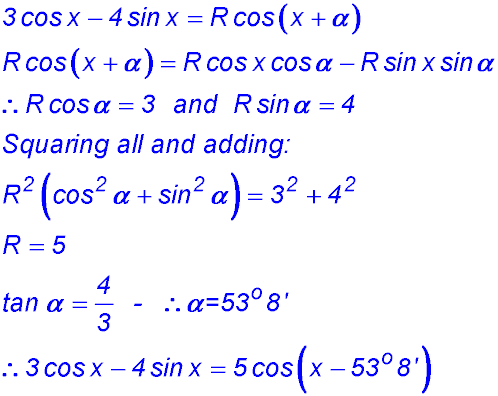(ii)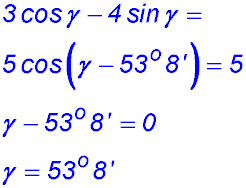11.12.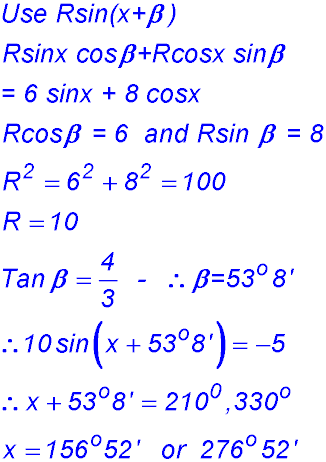13.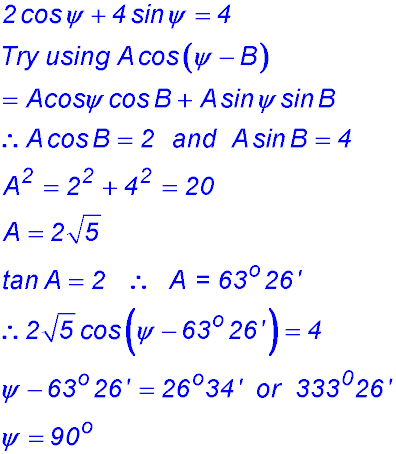14.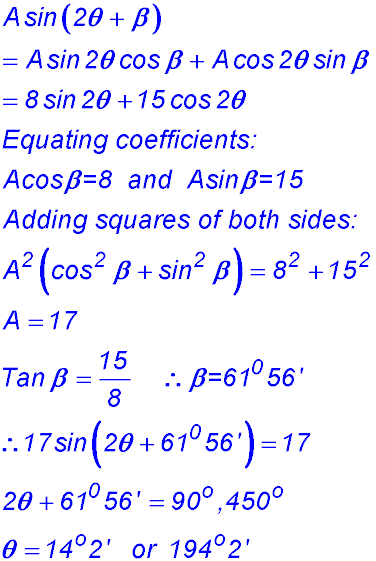15.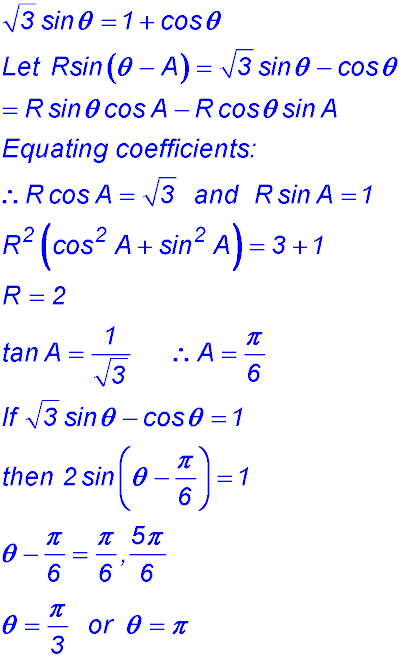16. (i)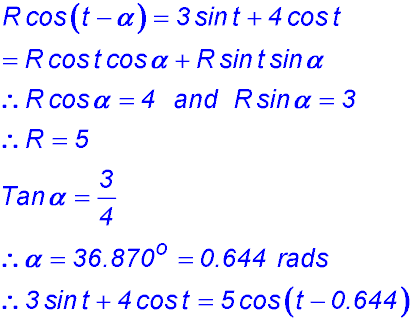(ii)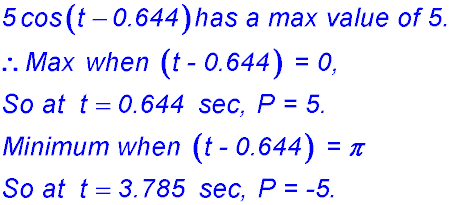17. (i)(ii) Initial height is 12 metres (= 13sin (1.176)). (iii)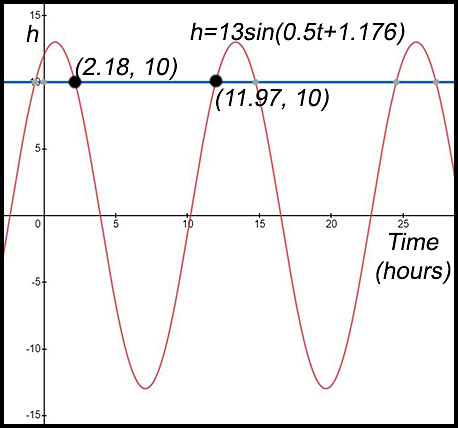18. (i)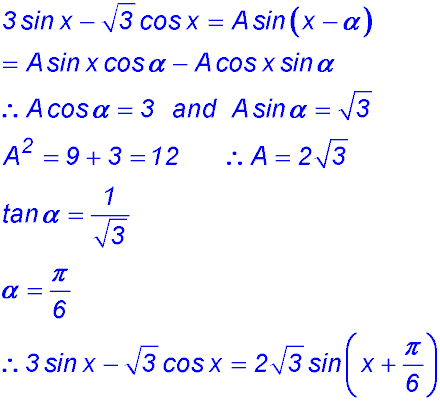(ii)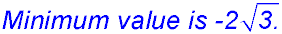(iii)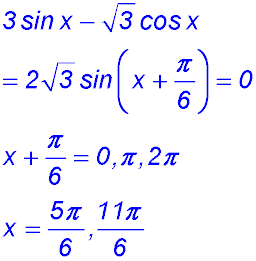19. (i)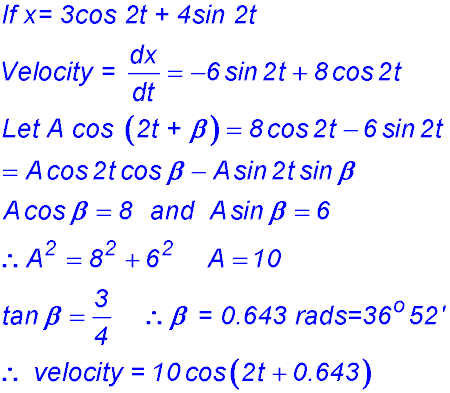(ii)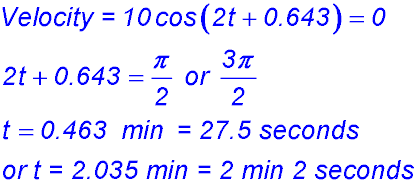20.